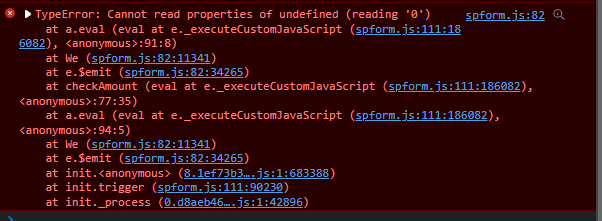# DataTable not saving to SharePoint when value is set by code

Hi,

I have a case where I enter a value in a specific cell and the rest of them are auto-populated. On beforeSave event I can see that the object value has all the inserted values but when I check the list the 2 auto-populated fields are not saved. If I try to enter the values manually then it works as expected.

Here is the code that triggers the auto-population:

``````fd.control('KitsDT').\$on('change', function (value) {
if (value) {
for (var i = 0; i < value.length; i++) {
if (_currentEditField === 'KitLotNumber') {
//kit lot number
let kitLotnumbers = kitsandreagents.filter(function (k) { return k.UpdatedValue === value[i].KitLotNumber });

if (kitLotnumbers.length > 0) {
value[i].KitBarcode = kitLotnumbers.Title;
value[i].KitExpiry = new Date(kitLotnumbers.SubmittedDate);
}
else {
value[i].KitExpiry = null;
}
}
else {
//kit bar code
let kitBarcodes = kitsandreagents.filter(function (k) { return k.Title === value[i].KitBarcode });

if (kitBarcodes.length > 0) {
value[i].KitLotNumber = kitBarcodes.UpdatedValue;
value[i].KitExpiry = kitBarcodes.SubmittedDate;
}
else {
value[i].KitExpiry = null;
}
}

}
}
fd.control('KitsDT').widget.refresh();
fd.control('KitsDT').saveState(value);

});
``````

Object Value before save:Saved data:

Do I do something wrong here?

Thanks,

George

Dear @george,
Small issue with the code - thank you for pointing it out! Just add this line `dt.\$emit('change');` for the change to be recorded in SharePoint field:

``````fd.control('KitsDT').\$emit('change');
fd.control('KitsDT').widget.refresh();
fd.control('KitsDT').saveState(value);``````
1 Like

Hi @Nikita_Kurguzov ,
Thank you for your piece of code, I had the same problem as mate @george ,
I did something similar without .saveState because I have this piece of code:

``````const checkAmount = (usrKilometer, usrCost) => {
const val = fd.control("dataKalkulace").value;
if (usrCost === 0 || usrKilometer === 0) {
fd.control("dataKalkulace").\$emit("change");
fd.control("dataKalkulace").widget.refresh();
return false;
}
if (usrKilometer <= 69) {
fd.control("dataKalkulace").\$emit("change");
fd.control("dataKalkulace").widget.refresh();
} else if (usrKilometer <= 99) {
fd.control("dataKalkulace").\$emit("change");
fd.control("dataKalkulace").widget.refresh();
} else if (usrKilometer <= 1000) {
fd.control("dataKalkulace").\$emit("change");
fd.control("dataKalkulace").widget.refresh();
}
};
``````

People can only use one row in the dataTable that is prepopulated with this code:

``````  const dTel = \$(fd.control("dataKalkulace").\$el);
// Define static Item to add
``````

I Also hide New Item and Trash can icon (ok in the picture you can see it).

The last two disabled automatically calculated fields did not want to be saved until I found out your code, but this error appears.Steo

Dear @Stepan,
Try removing different parts, see where the error comes from. saveState() is important to fix changes after they're applied, so you might also need it.

Dear @Nikita_Kurguzov ,
there should be the problem:

``````  const calculationMultiplication = (a, b) => {
return a * b;
};
const checkAmount = (usrKilometer, usrCost) => {
const val = fd.control("dataKalkulace").value;
if (usrCost === 0 || usrKilometer === 0) {
// fd.control("dataKalkulace").\$emit("change");
// fd.control("dataKalkulace").widget.refresh();
return false;
}
if (usrKilometer <= 69) {
// fd.control("dataKalkulace").\$emit("change");
// fd.control("dataKalkulace").widget.refresh();
} else if (usrKilometer <= 99) {
// fd.control("dataKalkulace").\$emit("change");
// fd.control("dataKalkulace").widget.refresh();
} else if (usrKilometer <= 1000) {
// fd.control("dataKalkulace").\$emit("change");
// fd.control("dataKalkulace").widget.refresh();
}
};

fd.control("dataKalkulace").\$on("change", function (val) {
fd.control("dataKalkulace").\$emit("change");
fd.control("dataKalkulace").widget.refresh();
fd.control("dataKalkulace").saveState(val);
});
});
``````

I think the variable "val" is not correct in this change, but cannot figure it out.
I only use one line (row) in data Table - every changes happen in that one row (first row).

Could you help me out?
Thank you
Steo

Dear @Stepan,
Can't see anything wrong, looking at it like that. Any errors in browser's console?

The post above is a screenshot from the browser.
Whenever I do a change and \$emit("change") and saveState triggers, the error appears in the console.

Although, I totally refactored the code and fields, so I have left the DataTable out and created several SharePoint one line text fields and do calculations among them.
So there is no problem at this moment, but thank you for your helpSteo

Dear @Stepan,
One thing you might need to make sure is that you're using the latest version of the app and the app package, find out how to update it for SharePoint 2019 here - Update Plumsail Forms On-Premises solution — SharePoint forms